### How to try to predict the output of Micali-Schnorr Generator (MS-DRBG) knowing the factorization. Part II

See  also Part I and Part III  of this series

tl;dr In the previous article of the same series we tried to predict the output of Micali-Schnorr Generator (MS-DRBG) knowing the factorization. In this blog post we continue the effort started in part I showing different strategies.  If you want to skip all my failures and go directly to the (in my humble opinion) most promising approach you can read directly the Solinas Prime and Generalized Mersenne Numbers section below.

If you actually wonder what is MS-DRBG and why I am trying to do it I'd suggest to go back and read the first article.

What I am NOT claiming in this post though is that there is a NSA's backdoor in the ANSI and ISO standards.

# Introduction and Failure #1

So let's start from were we actually finished the last post. We focused on an easier version of the problem directly extracted from the original Micali Schnorr paper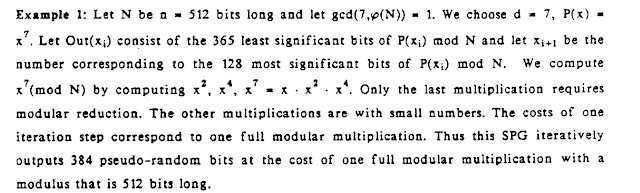from Efficient, perfect random number generators

where the known output is up to 3/4 of the RSA computation and secret state is only 1/4 of the RSA computation.

Assuming we know the factorization of N = p * q  we were using Crandall's primes in order to the least significant bits of (s)^e mod(p) and (s)^e mod (q) with s being the secret state at step i-1.
This at first sight looked cool and new to me but I quickly realized, having had some nice reviews and conversations (THANKS!!!), that this result was not too useful....

# Failure #2

This was the phase were I was thinking I was using techniques that are too trivial. All I described in the previous post is, at the end of the day, really trivial math that is known by any Computer Science student and if this problem has been open for so many years it MUST mean that I need to use some fancy stuff if I really want to solve this riddle. Right?
This happened at the same time of ROCA vulnerability that used some lattice sophisticated  attack first discovered by Coppersmith. So I tried, as hard as I could. Nothing came out from it....

# Failure #3

I have to admit I was really close to give up at this point, but I have decided to go a bit back on my path and review some of the assumptions I made hoping to find some caveats in my reasoning. If you remember one of the think I quickly dismissed previously was the usage of Mersenne primes (because the lack of the 2^128,2^520 interval, the one interesting for cryptography). This is actually a pity because Mersenne primes have some really beautiful properties. One peculiar one is that if you multiply a number x for 2^k modulo a Mersenne prime, this corresponds to nothing more than a left-circular shift by k bits:from "Prime Numbers: A Computational Perspective"

This is really interesting indeed. Why? Let's see.
Taking the same example reported in  Prime Numbers: A Computational Perspective
take N = 2^17 -1 =  131071 = 111111111111111112, x = 8977 = 100011000100012 and consider the product 2^5 * x (mod N). This will be the left-circular shift of x by 5 bits, or 25122 = 1100010001000102
• 2^17 * x (mod N) = x indeed  2^17 * 25122 (mod 131071) = 25122
• 2^(17*i) * x (mod N) = x for any integer i > 1
Be sure to keep this observations in mind and let's continue.  Another beautiful property of Mersenne primes is the following:from "Handbook of Elliptic and Hyperelliptic Curve Cryptography"
Basically reduction modulo a Mersenne prime is really trivial (See above).

### Playing with Mersenne primes and Chinese Remainder Theorem

Now you might ask, why those 2 Mersenne primes's properties are so interesting for  Micali-Schnorr Generator (MS-DRBG) ? Before to answer this question we need to open a parenthesis and talk about Chinese Remainder Theorem (CRT from now on). We already mentioned CRT in the previous post where I highlighted that, even I, successfully used CRT in a couple of crypto attacks  and . But we never talked about the CRT algorithm per se. The great property of the CRT algorithm is that is reasonably efficient and it is based on the idea of divide a big problem into many little problems. Then, once the little problems are solved, there is a reconstruction phase.
There are different methods for CRT but I would like to focus on one in particular (listed in "An Introduction to Mathematical Cryptography"):from "An Introduction to Mathematical Cryptography"

### Mersenne primitive part and cyclotomic polynomial

Now let's us put all the pieces together. We have seen that Mersenne primes have some beautiful properties and an algorithm for CRT. But as we know Mersenne primes are relatively scarce (specially in some interval as highlighted previously).  "Luckily" there are some other numbers (not all primes) that have the same "magic" properties:
Namely Mersenne primitive part and cyclotomic polynomial see below the  OEIS sequence (bonus track FWIW some years ago I managed to have my own little sequence listed there) .

This sequence contains an expansion of the first 1000 entries. But what can we do with those numbers?

#### Check for plaintext

Let's assume there are 2 distinct Mersenne primes : p=2^7-1 and q=2^14-1 (I know 2^14-1 is not a prime but please trust me and bear with me just a bit more).
As usual N = p * q and we know how to factorize. At step i we will have the MS-DRBG instance looking like (assuming s is the unknown internal state, and z the output of the generator):
M = s * 2^14 + z (mod N)
If we know p and q we  also know that :
M (mod p)= s * 2^14 (mod p) + z (mod p)
and
M (mod q) = s * 2^14 (mod q) + z (mod q)
Now s is still unknown but if we put all the things we learn so far is clear that s * 2^14 e(mod p) + z (mod p) = s + z (mod p)and  s * 2^14 (mod p) + z (mod q) = s + z (mod q) (do you remember this beautiful property of Mersenne primes?).
And following the CRT method seen above
s + zp + p*y  =  s + zq (mod q)
that is equal  to
zp + p*y  = zq (mod q)
Now given the fact gcd(p,q) = 1 we can calculate the value of y and eventually the most significant bits of R = M (mod p*q).
As we said 2^14-1 is not prime but we can easily replace this with cyclotomic polynomials that are not scarce at all.  E.g. using the 5th (31) and 25th (1082401) entry of the above list.

2^5 * x (mod 31) = x
but also
2^(5*5) * x (mod 31) = 2^(25) * x (mod 31) = x
but most importantly
2^(25) * x (mod 1082401) = x

So finally we have our p = 31 and q = 1082401 and we can apply the CRT trick seen above.

Let spell this down, we just got the most significant bits of M(mod p*q)without knowing the value of s. The problem is that the missing bits correspond to s itself sigh :(

# The night is darkest just before the dawn

What we got so far is not too useful again. Lets recap:
• In part I we managed to retrieve least significant bits of (s)^e mod(p) and (s)^e mod (q)
• in this post, so far we are able to guess the most significant bits of M(mod p*q)
Well it seems that we are screwed. If try to gain some information in one side we miss the other and viceversa :(

The effort so far can be summarized by this awesome image from Ange Albertini
So back to square one :(
I know we are already looking at an easier version of the problem but maybe we can be even less ambitious and lower even more the bar. Well it turns out that for this specific problem this is actually possible :) Indeed (as explained in a comment here) there is a prime-modulus variant that was introduced as an open problem at Micali and Schnorr, "Efficient, Perfect Polynomial Random Number Generators", chapter 4.from Efficient, perfect random number generators

But is this really easier?
I was basically stuck until something on the Wikipedia entry for Mersenne primes caught my attention:from Wikipedia

Where I heard this Solinas before? Oh of course,  Jerry Solinas is the NSA employee that authored the NIST curves and other stuff (*cough* Dual_EC_DRBG ? *cough*)... mmm interesting.

## Solinas Prime and Generalized Mersenne Numbers

So let's give a look to this Solinas prime.from Wikipedia
Cool, so we have a modular reduction algorithm that use linear feedback shift register. Interesting. Let's give a look to the original Solinas paper : Generalized Mersenne Numbers. So the example discussed in the paper shows a prime of the form:

p = 2^3k -2^k +1

The modular reduction obtained the linear feedback shift register is the followingfrom Generalized Mersenne Numbers
and here is the numerical example: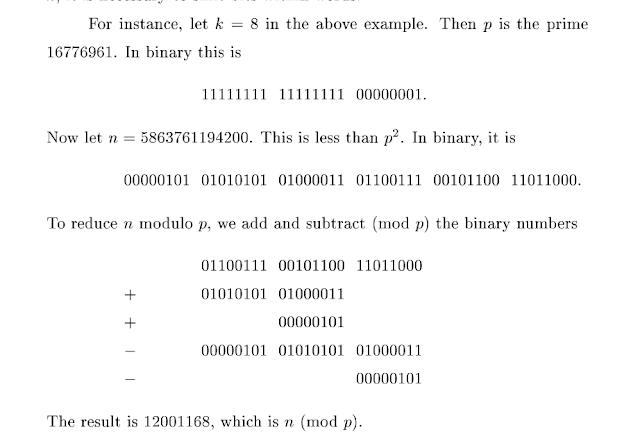from Generalized Mersenne Numbers
This is indeed really computationally efficient but it is not what we are interested at. When I looked at this the first question that came to my mind was: is the algorithm reversible? Namely is it possible given the result (or part of the result) obtain the original number? Well of course the answer is NO. But is it possible for some peculiar number. Well let's take in consideration a special example, where

• A5 = A4 = A2 and
• A0 = A3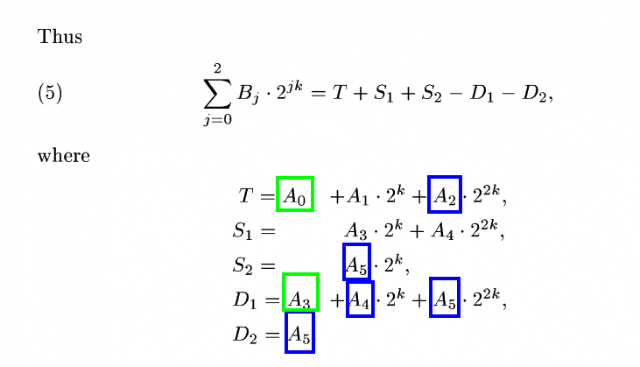from Generalized Mersenne Numbers

then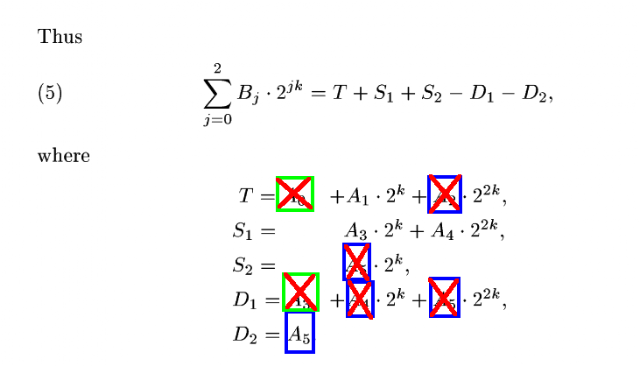from Generalized Mersenne Numbers

Hence
• B0 = -A5
• B1 = A1+A3
• B2 = A4 = A5
Nice.  If we try to map this to our original Micali-Schnorr problem this might be seen as:
• B0 + B1*2^k  is the output of the generator.
• B2 is the internal state (the one we want to recover).

Well it now turns out that for this particular instance of the problem it is possible to recover the internal state given the output. Indeed  B2 = A4 = A5

But we know -A5  = B0 so we can easily recover the state!!!
Now how likely is that we hit this number? Well a rough calculation says that the probability is really low :( and if I am not mistaken is roughly 1/2^3k. What is encouraging though is that we obtained this result from a NSA published paper that used one generalization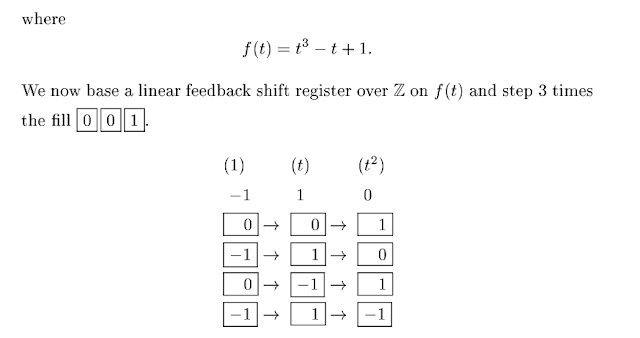from Generalized Mersenne Numbers

My true hope and OPEN QUESTION is that if exists another generalization that gives better result. I am truly convinced the numbers published in the ANSI X9.82 and ISO standards are following the same idea used in Solinas prime and my next goal is to work to prove it.

# Conclusions

In this blog post we again tried to predict the output of Micali-Schnorr Generator (MS-DRBG) knowing the factorization (and possible backdoor it). If any backdoor exists in the ANSI and ISO standards I think there is a possibility that the Solinas primes are used somehow.
My hope is, as always, that my work will inspire someone else to finally find the final solution for this great riddle. Any comment and critique is more than welcome!

# Acknowledgement

I would like to thank Matthew Green to give me such a good puzzle :) and  Nadia Heninger.

### OpenSSL Key Recovery Attack on DH small subgroups (CVE-2016-0701)

Usual Mandatory Disclaimer: IANAC (I am not a cryptographer) so I might likely end up writing a bunch of mistakes in this blog post... tl;dr The OpenSSL 1.0.2 releases suffer from a Key Recovery Attack on DH small subgroups . This issue got assigned CVE-2016-0701 with a severity of High and OpenSSL 1.0.2 users should upgrade to 1.0.2f. If an application is using DH configured with parameters based on primes that are not "safe" or not Lim-Lee (as the one in RFC 5114 ) and either Static DH ciphersuites are used or DHE ciphersuites with the default OpenSSL configuration (in particular SSL_OP_SINGLE_DH_USE is not set) then is vulnerable to this attack.  It is believed that many popular applications (e.g. Apache mod_ssl) do set the  SSL_OP_SINGLE_DH_USE option and would therefore not be at risk (for DHE ciphersuites), they still might be for Static DH ciphersuites. Introduction So if you are still here it means you wanna know more. And here is the thing. In my last bl

### Critical vulnerability in JSON Web Encryption (JWE) - RFC 7516

tl;dr if you are using go-jose , node-jose , jose2go , Nimbus JOSE+JWT or jose4j with ECDH-ES please update to the latest version. RFC 7516 aka JSON Web Encryption (JWE) hence many software libraries implementing this specification used to suffer from a classic Invalid Curve Attack . This would allow an attacker to completely recover the secret key of a party using JWE with Key Agreement with Elliptic Curve Diffie-Hellman Ephemeral Static (ECDH-ES) , where the sender could extract receiver’s private key. Premise In this blog post I assume you are already knowledgeable about elliptic curves and their use in cryptography. If not Nick Sullivan 's A (Relatively Easy To Understand) Primer on Elliptic Curve Cryptography or Andrea Corbellini's series Elliptic Curve Cryptography: finite fields and discrete logarithms are great starting points. Then if you further want to climb the elliptic learning curve including the related attacks you might also want to visit https://s

### The Curious Case of WebCrypto Diffie-Hellman on Firefox - Small Subgroups Key Recovery Attack on DH

tl;dr Mozilla Firefox prior to version 72 suffers from Small Subgroups Key Recovery Attack on DH in the WebCrypto 's API. The Firefox's team fixed the issue r emoving completely support for DH over finite fields (that is not in the WebCrypto standard). If you find this interesting read further below. Premise In this blog post I assume you are already knowledgeable about Diffie-Hellman over finite fields and related attacks. If not I recommend to read any cryptography book that covers public key cryptography. Here is a really cool simple explanation by David Wong : I found a cooler way to explain Diffie-Hellman :D pic.twitter.com/DlPvGwZbto — David Wong (@cryptodavidw) January 4, 2020 If you want more details about Small Subgroups Key Recovery Attack on DH I covered some background in one of my previous post ( OpenSSL Key Recovery Attack on DH small subgroups (CVE-2016-0701) ). There is also an academic pape r where we examine the issue with some more rigors.